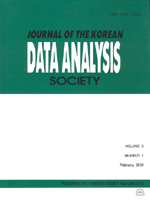상세검색
최근 검색어 전체 삭제
다국어입력
즐겨찾기0KCI등재 학술저널

# Bootstrap Prediction Interval estimator of SVM

• 등재여부 : KCI등재
• 2004.06
• 655 - 662 (8 pages)

Prediction interval estimation based on bootstrap method is presented for the support vector machine regressions, which allow us to perform even nonlinear regression by constructing a linear regression function in a high dimensional feature space. The bootstrap is applied to generate the bootstrap samples for the estimation of the covariance of the regression parameters consisting of the optimal bias and Lagrange multipliers. Experimental results are given which indicate the performance of proposed algorithm.

1. Introduction

2. Support Vector Machine Regression

3. Bootstrap Prediction Intervals

4. Numerical Studies

5. Conclusions and Remarks

References